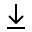MathBin Parametric Equations Member

A parametric equation defines a group of quantities as functions of one or more independent variables called parameters. Parametric equations usually express the coordinates of the points that make up a geometric object such as a curve (2D) or surface (3D), in which case the equations are collectively called a parametric representation or parameterization of the object. For example, the equations x = cos t and y = sin t form a parametric representation of the unit circle, where t is the parameter: A point (x, y) is on the unit circle if and only if there is a value of t such that these two equations generate that point. Sometimes the parametric equations for the individual scalar output variables are combined into a single parametric equation in vectors: (x, y) = (cos t, sin t).

Bins in Two Dimensions Special Parametric Curves
1. Astroid x2/3 + y2/3 = 1
2. Bicorn y2(a2 - x2) = (x2 + 2a y - a2)2
3. Cardioid (x2 + y2 - 2x)2 = 4a2(x2 + y2)
4. Cochleoid (x2 + y2) tan-1(yx) = a y
5. Circle x2 + y2 = 1